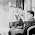Enoch Lai from en.wikipedia.org [GFDL or CC-BY-SA-3.0], via Wikimedia Commons

1. 所有簡單語句 $P_1, P_2, P_3, ...$ 都是合規式
2. 如果 $α$ 是合規式，則 $(∼α)$ 也是合規式
3. 如果 $α$ 和 $β$ 都是合規式，則 $(α∧β)$, $(α∨β)$, $(α→β)$, $(α↔β)$ 也是合規式。
4. 除了符合條件 1-3 的符號，其他全部不是合規式。

1. 簡單語句的左右括號數目一樣
2. 如果 $α$ 的左右括號數目一樣，則 $(∼α)$ 的左右括號數目一樣
3. 如果 $α$ 和 $β$ 的左右括號數目一樣，則 $(α∧β)$, $(α∨β)$, $(α→β)$, $(α↔β)$ 的左右括號數目也是一樣。

Base Case.
1. 由於簡單語句沒有左括號，也沒有右括號，左右括號數目都是 0 ，因此簡單語句的左右括號數目一樣。
Inductive Step.
1. 假設 $α$ 的左右括號數目一樣。 $(∼α)$ 的左括號數目是 $α$ 的左括號數目加 1 ，右括號數目是 $α$ 的右括號數目加 1 ，因此 $(∼α)$ 的左右括號數目一樣。
2. 假設 $α$ 和 $β$ 的左右括號數目一樣。令 $⋆$ 為任何一個二位連詞。 $(α⋆β)$ 的左括號數目等於 $α$ 的左括號數目加 $β$ 的左括號數目再加 1 ，右括號數目等於 $α$ 的右括號數目加 $β$ 的右括號數目再加 1 ，所以 $(α⋆β)$ 的左右括號數目一樣。

1. 最小的自然數 (通常是 0) 有性質 P
2. 如果自然數 n 有性質 P ，則 n+1 也有性質 P
────────────
/∴ 所有自然數都有性質 P

1. 對於每一個自然數 n ，如果所有小於 n 的自然數都有性質 P ，則 n 也有性質 P
────────────
/∴ 所有自然數都有性質 P

1. 所有簡單語句有性質 P
2. 如果合規式 $α$ 有性質 P ，則 $(∼α)$ 也有性質 P
3. 如果合規式 $α$ 和 $β$ 有性質 P ，則 $(α∧β)$, $(α∨β)$, $(α→β)$, $(α↔β)$ 也有性質 P
────────────
/∴ 所有合規式都有性質 P

（以證明 $∧$ 和 $→$ 不足以定義五個古典連詞。 ）

$sum(k) =_{df} 0+1+2+...+k$

$$sum(1)=\frac{1(1+1)}{2}$$
$$sum(2)=\frac{2(2+1)}{2}$$
$$sum(3)=\frac{3(3+1)}{2}$$

Base Case.
1. $sum(0)=0=\frac{0(0+1)}{2}$
Inductive Step.
1. 假設 $sum(k)=\frac{k(k+1)}{2}$ ，要證明 $sum(k+1)=\frac{(k+1)((k+1)+1)}{2}$。

\begin{split} sum(k+1) & = & sum(k)+(k+1) & \\ & = & \frac{k(k+1)}{2}+(k+1) & \text{(根據歸納假設)}\\ & = & \frac{{k(k+1)}+2(k+1)}{2} & \\ & = & \frac{(k+1)(k+2)}{2}& \end{split}

Bostock, David (1997). Intermediate Logic. Oxford University Press.
Causey, Robert (2006) Logic, Sets, and Recursion (2nd.). Jones and Bartlett Publishers.
Enderton, Herbert (1977). Elements of Set Theory. Academic Press, Inc.
Epstein, Richard & Carnielli, Walter (2000). Computability: Computable Functions, Logic, and the Foundations of Mathematics (2nd.). Wadsworth/Thomson Learning.
Hunter, Geoffrey (1973). Metalogic: An Introduction to the Metatheory of Standard First Order Logic. University of California Press.
Priest, Graham (2008). An Introduction to Non-Classical Logic (2nd.). Cambridge University Press.
Sider, Theodore (2010). Logic for Philosophy. Oxford University Press.

#### 7 則留言:

1.我學的倒是叫做「結構歸納法」(structural induction)，可用於遞歸定義的結構。而數學歸納法以及文中的歸納原則只是特例。如你所說，數學歸納法應用於自然數——應該是最簡單的遞歸定義結構，合規式則是另一種遞歸定義結構。

最近學後設邏輯，另一個例子就是字串。假設字母集是 A，可以如此定義 A 構成的字串︰

Base: 0（空字串）是一個字串。
Inductive rule: 假如X 是一個字串，y 是一個字母（y 是 A 的元素），則Xy（X連着y）也是一個字串。
Closure: 別無其他字串。

在只有一個字母的時候，結構基本上跟自然數一樣，數字則可表示成 0, a, aa, aaa, aaaa, ...。若有其他字母的話，通常使用結構歸納法比較簡單直接（對於所有字串 X，假如對任何字母y來說，Xy 都有某性質 P，則所有字串均有性質 P）。當然也可以像改寫成數學歸納法，對字串長度作歸納。

（寫到這兒才想起）用超限歸納法 (transfinite induction) 才能證明的命題，就是結構歸納法可以處理而數學歸納法無法處理的例子。

2.謝謝你的訊息。 Epstein & Carnielli 在 Computability 第十一章用的好像就是結構歸納法，不過他們沒有提這個名字，而直接叫做 simple induction 。（可能是我眼殘看不到）

多口一問，你有沒有推薦哪本後設邏輯的書，讓我學習學習

3.細想了一下，對形式系統最早的理解可能是來自 Gödel, Escher, Bach 一書（當然這本書不是為學邏輯而設）。實際上書讀得不多，反而主要靠上課。其實邏輯書很多時就是在講後設邏輯啊，不是從數學/邏輯根基研究出發的話，一般不會明確劃分。在 google books 看了 Hunter 那本的目錄，都應該涵蓋了不少東西。

短一點的可讀 Crossley 等人的 What is mathematical logic? 知個大概，Hamilton 的 Logic for mathematicians 我只是速讀（而且好像沒有讀完），你可看看是否合用。另外可試 Peter Smith 的 Introduction to Gödel's theorems，最近讀完，可謂大補丸（雖然題目專門了一點），有助釐清很多重要概念。Peter Smith 的 blog 也不錯，有很多學習材料，亦會寫點書評、推介好書之類︰http://www.logicmatters.net/。

要經典的話可選 S.C. Kleene 的 Introduction to metamathematics，我斷斷續續讀了三分一，非常紮實，頗多符號，不過可以速讀略去細節，有興趣的才細閱。

Model Theory 這學期才接觸，參考書有Chang & Keisler、Wilfrid Hodges 和 David Marker 的 Model Theory（三本書同名...），但主要還是靠課堂筆記。這科應該算是很技術性的科目，如不想迷失在符號中，看 Crossley 那本的簡介就好。

Computability theory 我幾乎完全不懂，只知道最基本的東西，就沒法推介了（Introduction to Gödel's theorems 最後有介紹圖靈機）。

4.昨晚留了個言，不知怎的不見了（可能是我多手關了 tab），簡略再答一次︰
書我讀得不多（相對來說上課學的更多），所以只能說自己讀過的。

J. N. Crossley 等人的 What is Mathematical Logic? 是本入門小書，簡介了若干重要題目，包括謂詞邏輯的完備性、模型論、圖靈機與遞歸函數、不完全定理和集合論。沒有太多技術細節，未必適合學習，但可以一覽各科的關聯。

最近讀了 Peter Smith 的 An Introduction to Gödel’s Theorems，非常詳細的介紹，也釐清很多概念，極之有用。此外 Peter Smith 寫了一些教材放上網，可以找來看看︰http://logicmatters.net/

S.C. Kleene 的 Introduction to metamathematics，據稱是經典，我只斷斷續續看了百多頁，很多符號，但不算辛苦。

5.剛剛才現原本的留言被 blogger 列入 spam ，現在已經搬了出來。

我只有修過大學＜基礎邏輯＞和一門研究所的＜邏輯與計算（上）＞，其他都是靠上網找清單來讀（例如 Peter Smith 那篇長得要死的 “Teach Yourself Logic”）。我原本是有些哲學目的才開始讀邏輯，後來看到有點上癮，胡亂找到就讀，所以還是想知道其他人看甚麼書學。你的推薦很實用，我會逐本慢慢磨，感謝！

6.哈，比我好了，我大學讀數學，只有第一年其中一門課介紹了最基本的集合論和邏輯，然後就沒有了。結果是幾年前有個 GEB 的讀書會，重讀一次（讀書會只讀了百多頁就停止），期間想去讀邏輯和數學哲學，才去找點書讀準備一下。幸運地在梅馨找到 Introduction to metamathematics，才第一次見 deduction theorem。（中學時有本朱水林的現代邏輯入門，介紹 Frege-Hilbert system，不過當時只見一大堆符號沒弄清楚發生甚麼事。）

7.原來如此。真沒想到梅馨會有 Introduction to metamathematics.....